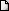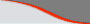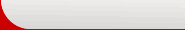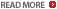CAT Math Preparation - Trigonometry, geometry. IIM, XAT, MBA Classes, study material, booksLATEST NEWS • Ascent Success Stories • TANCET 16 Classes @ Chennai • XAT 16 Classes @ ChennaiHome | TANCET Coaching Class | TANCET study material | Online TANCET Course | Contact us
 Ascent's Courses CAT 2010 for IIMs CAT Classroom Chennai Math & DI Crash Course CAT - Correspondence CAT Correspondence India CAT Corres Abroad Mock CAT Series CAT Crash Course Math Shortcut Workshops XAT Classes TANCET 2010 Classes MAT Classes GRE-GMAT GMAT Classes US B Schools Faculty List Question A Day Business Partnership Jobs @ Ascent Testimonials Ascent TANCET Toppers### Number theory / Trigonometry - Quant/Math - CAT 2016

Question 4 the day: June 28, 2002

The question for the day is an interesting number systems / trigonometry problem.

Question:
'a' and 'b' are the lengths of the base and height of a right angled triangle whose hypotenuse is 'h'. If the values of 'a' and 'b' are positive integers, which of the following cannot be a value of the square of the hypotenuse?
(1) 13
(2) 23
(3) 37
(4) 41
Correct choice (2). Correct Answer - 23

Solution:

The value of the square of the hypotenuse = h2 = a2 + b2

As the problem states that 'a' and 'b' are positive integers, the values of a2 and b2 will have to be perfect squares. Hence we need to find out that value amongst the four answer choices which cannot be expressed as the sum of two perfect squares.

Choice 1 is 13. 13 = 9 + 4 = 32 + 22. Therefore, Choice 1 is not the answer as it is a possible value of h2

Choice 2 is 23. 23 cannot be expressed as the sum two numbers, each of which in turn happen to be perfect squares. Therefore, Choice 2 is the answer.

## XLRI XAT TANCET Practice Question Samples

#### Question Archives

GMAT Math Books
 Comprehensive study material for GMAT Quant. 1800+ questions.Math Lesson Book : GMAT CAT
 Math lesson book on Arithmetic, Algebra, Geometry, Data Sufficiency including Number Theory, Permutation and Combination. Plus Speed Tests.TANCET Diagnostic Test

 GMAT Math Lesson Book | SAT prep | GRE Classes @ Chennai | GMAT Coaching @ Chennai | TANCET Mailing List © 2002 - 17 ASCENT Education all rights reserved. Terms & Condition | Privacy Policy